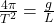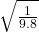## Harmonic Motions of Similar Models. The unifying power of mathematical methods results to a large extent from the fact that different physic

Question

Harmonic Motions of Similar Models. The unifying power of mathematical methods results to a large extent from the fact that different physical (or other) systems may have the same or very similar models. Illustrate this for the following three systems (a) Pendulum clock. A clock has a 1-meter pendulum. The clock ticks once for each time the pendulum completes a full swing, returning to its original position. How many times a minute does the clock tick

in progress 0
5 months 2021-08-15T13:59:00+00:00 1 Answers 26 views 0

## Answers ( )

tick = 30

Explanation:

The angular velocity in a simple pendulum is

w² = g / L

the angular velocity is related to the period

w = 2π/ T

let’s substituteT =indicate that the length is m = 1, if we assume that the acceleration of gravity is g = 9.8 m / s²

T = 2πT = 2.0 s

the clock has a tick each period and since the period is 2.0 s, to complete the minute (60 s) you need

tick = 1 60 / T

tick = 1 60/2

tick = 30